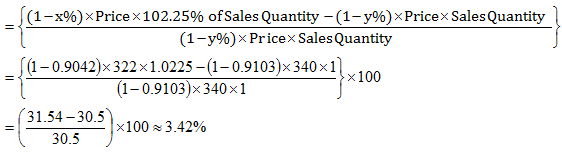Answer question nos. 1-3 based on the data given in table 1:
Cost and price data for Portland cement manufactured by Paharpur Cement and Bahsin Cement, for four consecutive quarters, are given in table 1:
Table 1: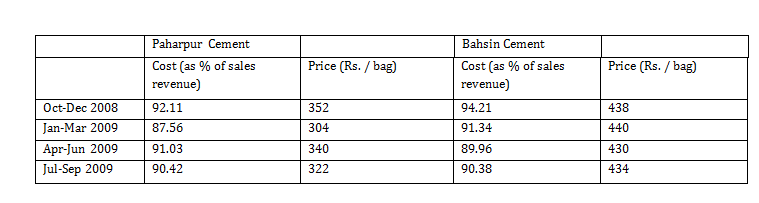Sales Revenue = Price × Sales Quantity

Profit = Sales Revenue – Cost

Profit Rate = Profit / Sales Quantity

Question 1. Profit rate of Paharpur Cement is more than the profit rate of Bahsin Cement in:
A. Oct-Dec 2008 and Jan-Mar 2009
B. Jan-Mar 2009 and Apr-Jun 2009
C. Oct-Dec 2008 only
D. Jan-Mar 2009 only
E. Apr-Jun 2009 only

Question 2. If between Jan-Mar 2009 and Apr-Jun 2009 sales of Paharpur Cement increased from 543278 to 698236 and that of Bahsin Cement decreased from 526532 to 499874, then which of the following is true?
A. Between Jan-Mar 2009 and Apr-Jun 2009, profit and profit rate of Paharpur Cement increased, whereas profit and profit rate of Bahsin Cement decreased.
B. Between Jan-Mar 2009 and Apr-Jun 2009, profit rate of Paharpur Cement increased but its profit decreased, whereas both profit and profit rate of Bahsin Cement increased.
C. Between Jan-Mar 2009 and Apr-Jun 2009, both profit and profit rate of Paharpur Cement decreased, whereas profit rate of Bahsin Cement decreased but its profit increased.
D. Between Jan-Mar 2009 and Apr-Jun 2009, profit of Paharpur Cement increased but its profit rate decreased, whereas profit rate of Bahsin Cement increased but its profit decreased.
E. Between Jan-Mar 2009 and Apr-Jun 2009, profit rate of Paharpur Cement decreased but its profit increased, whereas both profit and profit rate of Bahsin Cement increased.

Question 3. If between Apr-Jun 2009 and Jul-Sept 2009 sales of Paharpur Cement increased by 2.25%, it profit increased by
A. 2.08%
B. 2.25%
C. 2.96%
D. 3.28%
E. 3.42%

During , Oct-Dec 2008, we have

For Paharpur cement,

Revenue = 352 × x (where x is the number of bags)

Cost incurred = 0.9211 × 352 × x

Profit Rate =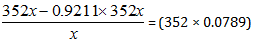For Bahsin cement, Profit rate = (438 × 0.0579)

During Jan-March 2009 profit rate for:

Paharpur cement = (304 × 0.1244)

Bahsin cement = (440 × 0.0866)

During April-June 2009 profit rate for:

Paharpur cemnt = 340 × 0.0897

Bahsin cement = 430 × 0.1004

For Paharpur cement

Revenue in Jan – Mar 2009 = 304 × 543278

Revenue in Apr – June 2009 = 340 × 698236

Profit rate for Jan – Mar 2009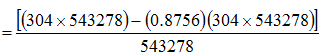……..(i)

Profit rate for Jan – Mar 2009

= 304(1– 0.8756) = 37.575                                                                                          ..……(ii)

Profit for Apr – June 2009 = 340 × 698236 – 0.9103 × 340 × 698236                      ….…..(iii)

Profit rate = (1 – 0.9103) × 340 = 30.498                                                                 ………(iv)

For Bahsin cement

Revenue in Jan-Mar 2009 = 440 × 526532

Revenue in Apr-June 2009 = 430 × 499874

Profit in Jan-Mar 2009 = 440 × 526532 – 0.9134 × 440 × 526532                              ………..(v)

Profit rate = 440 – 0.9134 × 440 = 38.104                                                                  ……….(vi)

Profit in Apr-Jun 2009 = 430 × 499874 – 0.8996 × 499874 × 430                              ……….(vii)

Profit rate = 430 – 0.8996 × 430 = 43.172                                                                  ………..(viii)

Checking through the profit rate we see that option (E) is the correct choice.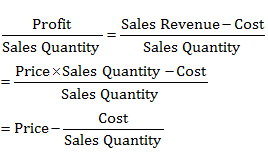Cost = x% of Sales Revenue = x% of (Price × Sales Quantity)

∴   Profit Revenue = Price – x% of Price

= (1 – x%) of Price

⇒ Profit = Profit Revenue × Sales Quantity

= (1 – x%) Price × Sales Quantity

∴   Increase in profit